Class 10 RD Sharma Solutions – Chapter 5 Trigonometric Ratios – Exercise 5.1 | Set 1

• Last Updated : 07 Dec, 2021

(i) sinA = 2/3

Solution:

sinA = 2/3 = Perpendicular/Hypotenuse

Draw a right-angled △ABC in which ∠B is = 90°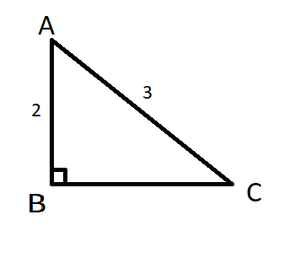Using Pythagoras Theorem, in △ABC,

(Hypotenuse)2 = (Perpendicular)2 + (Base)2

AC2 = AB2 + BC2

(3)2 = (2)2 + (BC)2

9 = 4 + BC

BC2 = 9 – 4 = 5

BC = √5 units

Now,

cosA = Base/Hypotenuse = BC/AC = √5/3

tanA = Perpendicular/Base = AB/BC = 2/√5

cotA = 1/tanA = √5/2

secA = 1/cosA = 3/√5

cosecA = 1/sinA = 3/2

(ii) cosA = 4/5

Solution:

cosA = 4/5 = Base/Hypotenuse

Draw a right-angled △ABC in which ∠B is = 90°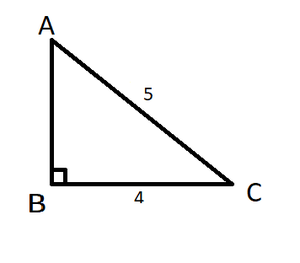Using Pythagoras Theorem, in △ABC,

(Hypotenuse)2 = (Perpendicular)2 + (Base)2

AC2 = AB2 + BC2

(5)2 = (AB)2 + (4)2

25 = AB2 + 16

AB2 = 25 – 16 = 9

AB = √9

= 3 units

Now,

sinA = Perpendicular/Hypotenuse = AB/AC =3/5

tanA = Perpendicular/Base = AB/BC = 3/4

cotA = 1/tanA = 4/3

secA = 1/cosA = 5/4

cosecA = 1/sinA =5/3

(iii) tanθ = 11/1

Solution:

tanθ = 11/1 = Perpendicular/Base

Draw a right-angled △ABC in which ∠B is = 90°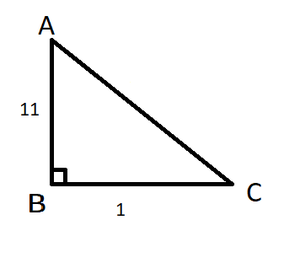Using Pythagoras Theorem, in △ABC,

(Hypotenuse)2 = (Perpendicular)2 + (Base)2

AC2 = AB2 + BC2

AC2 = (11)2 + (1)2

AC2 = 121  + 1

= 122

AC = √122units

Now,

sinθ = Perpendicular/Hypotenuse = AB/AC = 11/ √122

cosθ = Base/Hypotenuse = BC/AC = 1/√122

cotθ = 1/tanθ = 1/11

secθ = 1/cosθ = √122/1

cosecθ = 1/sinθ = √122/11

(iv) sinθ = 11/15

Solution:

sinθ = 11/15 = Perpendicular/Hypotenuse

Draw a right-angled △ABC in which ∠B is = 90°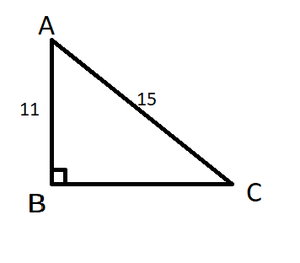Using Pythagoras Theorem, in △ABC,

(Hypotenuse)2 = (Perpendicular)2 + (Base)2

AC2 = AB2 + BC2

(15)2 = (11)2 + (BC)2

225 = 121 + (BC)2

(BC)2 = 104

BC = 2√26

Now,

cosθ = Base/Hypotenuse = BC/AC = 2√26/15

tanθ = AB/BC = 11/ 2√26

cotθ = 1/tanθ = 2√26/11

secθ = 1/cosθ = 15/ 2√26

cosecθ = 1/sinθ = 15/11

(v) tan α = 5/12

Solution:

tan α = 5/12 = Perpendicular/Base

Draw a right-angled △ABC in which ∠B is = 90°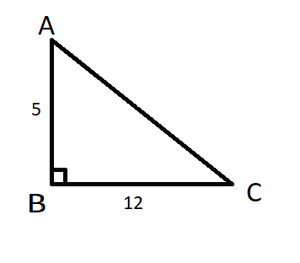Using Pythagoras Theorem, in △ABC,

(Hypotenuse)2 = (Perpendicular)2 + (Base)2

AC2 = AB2 + BC2

(AC)2 = (12)2 + (25)2

(AC)2 = 144 + 25

(AC)2 = 169

AC = √169 = 13 units

Now,

sin α = Perpendicular/Hypotenuse = AB/AC = 5/13

cos α = Base/Hypotenuse = BC/AC = 12/13

cot α = 1/tan α = 12/5

sec α = 1/cos α = 13/12

cosec α = 1/sin α = 13/5

(vi) sinθ = √3/2

Solution:

sinθ = √3/2 = Perpendicular/Hypotenuse

Draw a right-angled △ABC in which ∠B is = 90°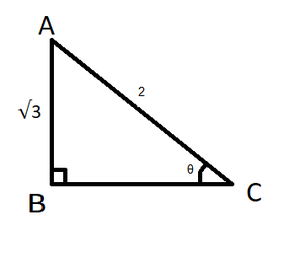Using Pythagoras Theorem, in △ ABC,

(Hypotenuse)2 = (Perpendicular)2 + (Base)2

AC2 = AB2 + BC2

(2)2 = (√3​)2 + (BC)2

4 = 3 + (BC)2

(BC)2 = 4 – 3 = 1

BC = 1 units

Now,

cosθ = Base/Hypotenuse = BC/AC = 1/2

tanθ = AB/BC = √3/1

cotθ = 1/tanθ = 1/√3

secθ = 1/cosθ = 2/1

cosecθ = 1/sinθ = 2/√3

(vii) cosθ = 7/25

Solution:

cosθ = 7/25 = Base/Hypotenuse

Draw a right-angled △ABC in which ∠B is = 90°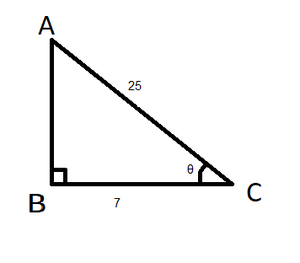Using Pythagoras Theorem, in △ ABC,

(Hypotenuse)2 = (Perpendicular)2 + (Base)2

AC2 = AB2 + BC2

(25)2 = (AB)2 + (7)2

625 = (AB)2 + 49

(AB)2 = 625 – 49 = 576

AB = √576 = 24 units

Now,

sinθ = Perpendicular/Hypotenuse = AB/AC = 24/25

tanθ = Perpendicular/Base = AB/BC = 24/7

cotθ = 1/tanθ = 7/24

secθ = 1/cosθ = 25/7

cosecθ = 1/sinθ = 25/24

(viii) tanθ = 8/15

Solution:

tanθ = 8/15 = Perpendicular/Base

Draw a right-angled △ABC in which ∠B is = 90°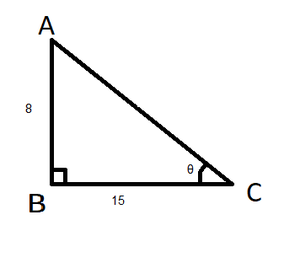Using Pythagoras Theorem, in △ ABC,

(Hypotenuse)2 = (Perpendicular)2 + (Base)2

AC2 = AB2 + BC2

(AC)2 = (8)2 + (15)2

(AC)2 = 64 + 225

AC = √289 = 17

Now,

sinθ = Perpendicular/Hypotenuse = AB/AC = 8/17

cosθ = Base/Hypotenuse = BC/AC = 15/17

cotθ = 1/tanθ = 15/8

secθ = 1/cosθ = 17/15

cosecθ = 1/sinθ = 17/8

(ix) cotθ = 12/5

Solution:

cotθ = 12/5 = Base/Perpendicular

Draw a right-angled △ABC in which ∠B is = 90°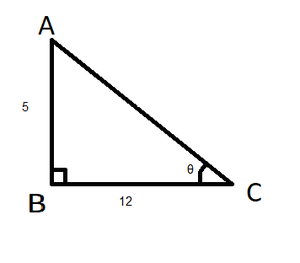Using Pythagoras Theorem, in △ABC,

(Hypotenuse)2 = (Perpendicular)2 + (Base)2

AC2 = AB2 + BC2

(AC)2 = (5)2 + (12)2

(AC)2 = 25 + 144

(AC)2 = 169

AC = √169 = 13 units

Now,

sinθ = Perpendicular/Hypotenuse = AB/AC = 5/13

cosθ = Base/Hypotenuse = BC/AC = 12/13

tanθ = 1/tanθ = 5/12

secθ = 1/cosθ = 13/12

cosecθ = 1/sinθ = 13/5

(x) secθ = 13/5

Solution:

secθ = 13/5 = Hypotenuse/Base

Draw a right-angled △ABC in which ∠B is = 90°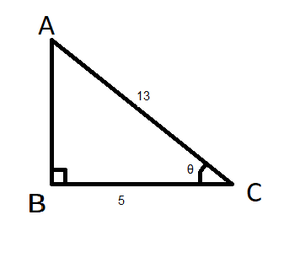Using Pythagoras Theorem, in △ABC,

(Hypotenuse)2 = (Perpendicular)2 + (Base)2

AC2 = AB2 + BC2

(13)2 = (AB)2 + (5)2

169 = (AB)2 + 25

(AB)2 = 169 – 25 = 144

AB = √144 = 12 units

Now,

sinθ = Perpendicular/Hypotenuse = AB/AC = 12/13

tanθ = Perpendicular/Base = AB/BC = 12/5

cotθ = 1/tanθ = 5/12

cosθ = 1/secθ = 5/13

cosecθ = 1/sinθ = 13/12

(xi) cosecθ = √10

Solution:

cosecθ = √10/1 = Hypotenuse/Perpendicular

Draw a right-angled △ABC in which ∠B is = 90°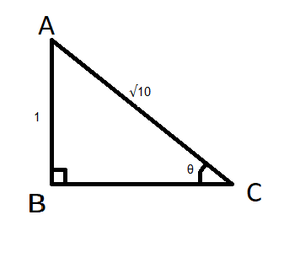Using Pythagoras Theorem, in △ ABC,

(Hypotenuse)2 = (Perpendicular)2 + (Base)2

AC2 = AB2 + BC2

(√10)2 = (1)2 + (BC)2

10 = 1 + (BC)2

(BC)2 = 10 – 1 = 9

BC = √9 = 3

Now,

sinθ = Perpendicular/Hypotenuse = AB/AC = 1/√10

cosθ = Base/Hypotenuse = BC/AC = 3/√10

tanθ = Perpendicular/Hypotenuse = AB/BC = 1/3

cotθ = 1/tanθ = 3/1 = 3

secθ = 1/cosθ = √10/3

(xii) cosθ = 12/15

Solution:

cosθ = 12/15 = Base/Hypotenuse

Draw a right-angled △ABC in which ∠B is = 90°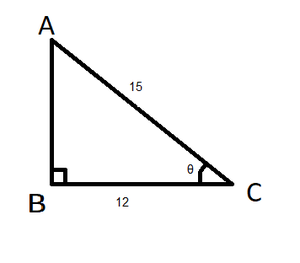Using Pythagoras Theorem, in △ABC,

(Hypotenuse)2 = (Perpendicular)2 + (Base)2

AC2 = AB2 + BC2

(15)2 = (AB)2 + (12)2

225 = (AB)2 + 144

(AB)2 = 225 – 144 = 81

AB = √81 = 9 units

Now,

sinθ = Perpendicular/Hypotenuse = AB/AC = 9/15

tanθ = Perpendicular/Base = AB/BC = 9/12

cotθ = 1/tanθ = 12/9

secθ = 1/cosθ = 15/12

cosecθ = 1/sinθ = 15/9

(ii) sin C, cos C

Solution:

Given:

In right-angled ΔABC,

AB = 24 cm, BC = 7 cm. ∠B = 90°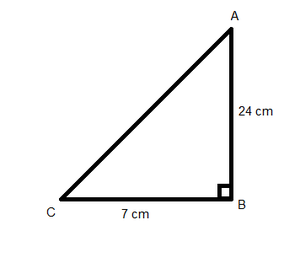Using Pythagoras Theorem

AC2 = AB2 + BC2

AC2 = 242 + 72 = 576 + 49

AC2 = 625

AC = √625 = 25cm

Now,

(i) sinA = BC/AC = 7/25

cosA = AB/AC = 24/25

(ii) sinC = AB/AC = 24/25

cosC = BC/AC = 7/25

Question 3. In the figure, find tan P and cot R. Is tan P = cot R?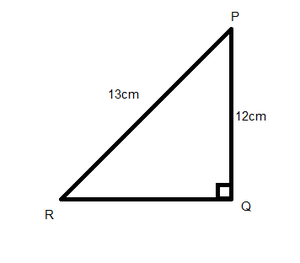Solution:

Using Pythagoras Theorem

PR2 = PQ2 + QR2

132 = 122 + QR2

QR2 = 169 – 144 = 25

QR = √25 = 5 cm

Now,

tan P = Perpendicular/Base = QR/PQ = 5/2

cot R = Base/Perpendicular = QR/PQ = 5/2

Yes, tanP = cot R

Question 4. If sin A = 9/41, compute cos A and tan A.

Solution:

Given, sinA = 9/41 = Perpendicular/Hypotenuse

Draw a △ ABC where ∠B = 90°, BC = 9, AC = 41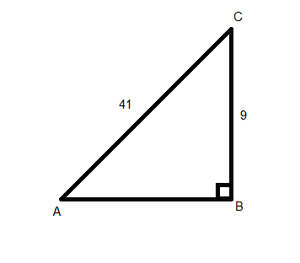Using Pythagoras Theorem

AC2 = AB2 + BC2

BC2 = 412 – 92 = 1681 – 81

BC2 = 1600

BC = √1600 = 40

Now, cos A = Base/Hypotenuse = AB/AC = 40/41

tan A = Perpendicular/Base = BC/AB = 9/40

Question 5. Given 15 cot A = 8, find sin A and sec A.

Solution:

Given, 15 cot A = 8

cot A = 8/15 = Base/Perpendicular

Draw a △ ABC where ∠B = 90°, AB = 8, BC = 15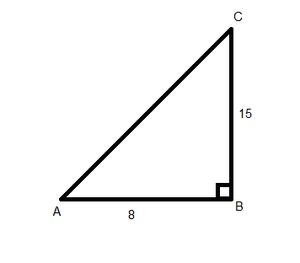Using Pythagoras Theorem

AC2 = AB2 + BC2

AC2 = 82 + 152 = 64 + 225

AC2 = 289

AC = √289 = 17

Now,

sin A = Perpendicular/Hypotenuse = BC/AC = 15/17

sec A = Hypotenuse/Base = AC/AB = 17/8

Question 6. In ΔPQR, right-angled at Q, PQ = 4 cm and RQ = 3 cm. Find the values of sin P, sin R, sec P, and sec R.

Solution:

In right-angled ΔPQR,

∠Q = 90°, PQ = 4cm, RQ = 3cm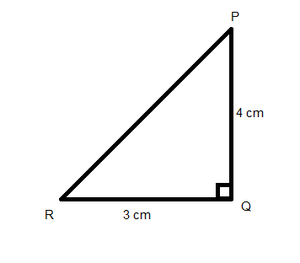Using Pythagoras Theorem

PR2 = PQ2 + QR2

PR2 = 42 + 32 = 16 + 9

PR2 = 25

PR = √25 =5

Now,

sin P = Perpendicular/Hypotenuse = RQ/PR = 3/5

sin R = Perpendicular/Hypotenuse = PQ/PR = 4/5

sec P = Hypotenuse/Base = PR/PQ = 5/4

sec R = Hypotenuse/Base = PR/RQ = 5/3

My Personal Notes arrow_drop_up